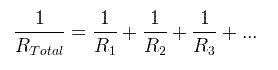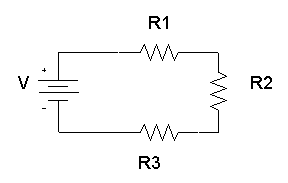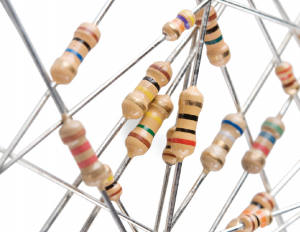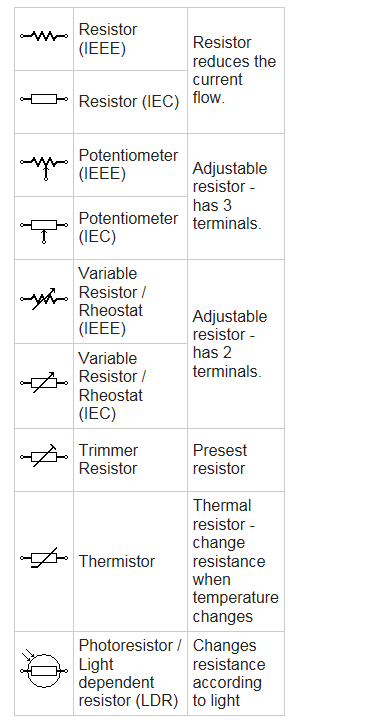# Resistor in LED Indicator Light

### What is resistor

Resistor is an electrical component that reduces the electric current.

The resistor’s ability to reduce the current is called resistance and is measured in units of ohms (symbol: Ω).

If we make an analogy to water flow through pipes, the resistor is a thin pipe that reduces the water flow.

### Ohm’s law

The resistor’s current I in amps (A) is equal to the resistor’s voltage V in volts (V)

divided by the resistance in ohms (Ω):

I=V/R

The resistor’s power consumption P in watts (W) is equal to the resistor’s current I in amps (A)

times the resistor’s voltage V in volts (V):

P=I*V

The resistor’s power consumption P in watts (W) is equal to the square value of the resistor’s current I in amps (A)

times the resistor’s resistance R in ohms (Ω):

P=I*R

The resistor’s power consumption P in watts (W) is equal to the square value of the resistor’s voltage V in volts (V)

divided by the resistor’s resistance R in ohms (Ω):

P=V/R

### Resistors in parallel

The total equivalent resistance of resistors in parallel RTotal is given by:So when you add resistors in parallel, the total resistance is decreased.

### Resistors in seriesThe total equivalent resistance of resistors in series Rtotal is the sum of the resistance values:

Rtotal = R1R2R3+…

So when you add resistors in series, the total resistance is increased.

### Dimensions and material affects

The resistance R in ohms (Ω) of a resistor is equal to the resistivity ρ in ohm-meters (Ω∙m) times the resistor’s length l in meters (m) divided by the resistor’s cross sectional area A in square meters (m2):

### Resistor image## Resistor symbols### Resistor color code

The resistance of the resistor and its tolerance are marked on the resistor with color code bands that denotes the resistance value.

There are 3 types of color codes:

4 bands: digit, digit , multiplier, tolerance.

5 bands: digit, digit, digit , multiplier, tolerance.

6 bands: digit, digit, digit , multiplier, tolerance, temperature coefficient.

#### Resistance calculation of 4 bands resistor

R = (10×digit+ digit2) × multiplier

#### Resistance calculation of 5 or 6 bands resistor

R = (100×digit+ 10×digit2+digit3) × multiplier